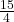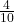Question

A rabbit’s fur grows at the rate of 5 over 2 inches per month.

How many months will it take the rabbit’s fur to grow 15 over 4 inches?

1.Euphemia
1.5 months
Step-by-step explanation:
First, makeso it has a common demoninator as.
We need the demoninator to be the same so we can multiplyby 2 to make it.
So, a rabbit’s ear growsof an inch in a month.
To find how many months, it will take, we have to divideby.
To divide fractions, we can multiply with the reciprocal.
So,*iswhich reduces towhich, in decimal form is equal to 1.5 months.

2.trungdung22
Growth rate = 5/2 inches per month
The fur length after y months:
Fur length = 5/2 × y
Therefore, to have a fur length of 15/4 inches :
15/4 = 5/2 × y
3.75 = 2.5y
Divide both sides by 2.5 to isolate y
3.75/2.5 = y
1.5 = y
it will take a month and half to have a fur of 15/4 inch length